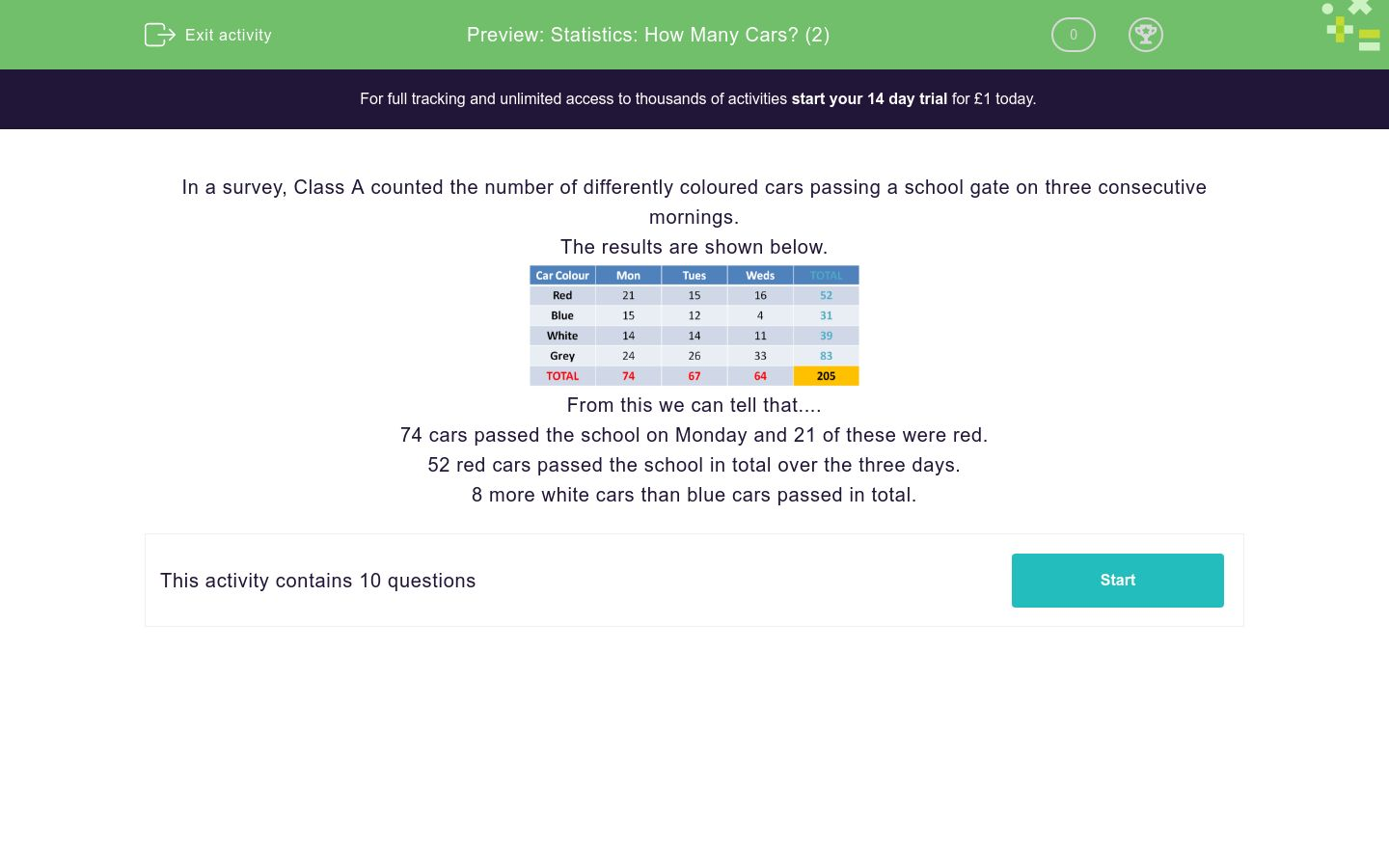# Statistics: How Many Cars? (2)

In this worksheet, students answer questions using the results of a survey shown in a data table.Key stage:  KS 2

Curriculum topic:  Statistics

Curriculum subtopic:  Compare Data Using Charts and Graphs

Difficulty level:### QUESTION 1 of 10

In a survey, Class A counted the number of differently coloured cars passing a school gate on three consecutive mornings.

The results are shown below.From this we can tell that....

74 cars passed the school on Monday and 21 of these were red.

52 red cars passed the school in total over the three days.

8 more white cars than blue cars passed in total.

Use the table of results to answer the question.

Which colour car passed most often on Monday?Use the table of results to answer the question.

Which colour car passed most often over the three days?Use the table of results to answer the question.

Which colour car passed least often in total?Use the table of results to answer the question.

How many cars passed on Tuesday?Use the table of results to answer the question.

How many non-white cars passed on Tuesday?Use the table of results to answer the question.

How many grey  and white cars passed in total over Monday and Tuesday?Use the table of results to answer the question.

How many non-red cars passed in total on Wednesday?Use the table of results to answer the question.

How many fewer grey cars passed  on Monday than on Wednesday?Use the table of results to answer the question.

Which colour car passed least often on Wednesday?Use the table of results to answer the question.

How many more grey cars than red cars passed in total over Monday and Wednesday?• Question 1

Use the table of results to answer the question.

Which colour car passed most often on Monday?CORRECT ANSWER
Grey
EDDIE SAYS
Read the table carefully. The results on Monday can be read vertically so the car that passed most often was Grey at 24 times.
• Question 2

Use the table of results to answer the question.

Which colour car passed most often over the three days?CORRECT ANSWER
Grey
EDDIE SAYS
Compare the results carefully over the three days. On each day the car that passed most often and had the highest total of passings was Grey at 83.
• Question 3

Use the table of results to answer the question.

Which colour car passed least often in total?CORRECT ANSWER
Blue
EDDIE SAYS
The words least often mean the smallest number of times passed. When looking at the table, it looks like the Blue car passed the least often as the total was 31.
• Question 4

Use the table of results to answer the question.

How many cars passed on Tuesday?CORRECT ANSWER
67
EDDIE SAYS
If you look at Tuesday's results, the red number at the bottom shows the total cars that passed that day - 67.
• Question 5

Use the table of results to answer the question.

How many non-white cars passed on Tuesday?CORRECT ANSWER
53
EDDIE SAYS
To solve this problem, look at Tuesday's results. Subtract the white cars from the total cars to get your answer: 67 - 14 = 53.
• Question 6

Use the table of results to answer the question.

How many grey  and white cars passed in total over Monday and Tuesday?CORRECT ANSWER
78
EDDIE SAYS
Add up the grey and white cars on Monday and Tuesday: 14 + 14 + 24 + 26 = 78. Well done!
• Question 7

Use the table of results to answer the question.

How many non-red cars passed in total on Wednesday?CORRECT ANSWER
48
EDDIE SAYS
To find how many non-red cars passed in total on Wednesday, subtract the red cars from the total to get your answer: 64 - 16 = 48. Good work!
• Question 8

Use the table of results to answer the question.

How many fewer grey cars passed  on Monday than on Wednesday?CORRECT ANSWER
9
EDDIE SAYS
Subtract the grey cars from Wednesday and Monday to get your answer: 33 - 24 = 9.
• Question 9

Use the table of results to answer the question.

Which colour car passed least often on Wednesday?CORRECT ANSWER
Blue
EDDIE SAYS
Look at Wednesday's results and find that the colour car that passed least often was Blue.
• Question 10

Use the table of results to answer the question.

How many more grey cars than red cars passed in total over Monday and Wednesday?CORRECT ANSWER
20
EDDIE SAYS
To solve this problem, you need to add the grey cars on Monday and Wednesday, as well as the red cars on Monday and Wednesday. Then subtract these totals to get your answer: (24 + 33) - (21 + 16) = 57 - 37 = 20. Well done!
---- OR ----

Sign up for a £1 trial so you can track and measure your child's progress on this activity.

### What is EdPlace?

We're your National Curriculum aligned online education content provider helping each child succeed in English, maths and science from year 1 to GCSE. With an EdPlace account you’ll be able to track and measure progress, helping each child achieve their best. We build confidence and attainment by personalising each child’s learning at a level that suits them.

Get startedStart your £1 trial today.
Subscribe from £10/month.